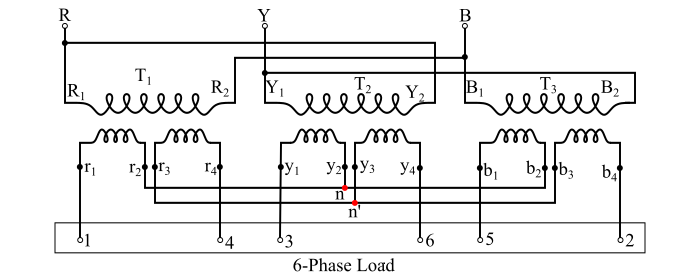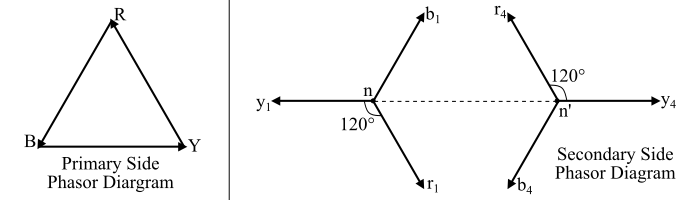# Double-Star Connection of Transformers – Three-to-Six Phase Transformation

The rectifiers are used to convert alternating current (AC) to direct current (DC). The advantages of increased number of phases of the AC supply are given as follows −

• A smoother waveform is obtained on the DC side as the number of phases of the AC supply is increased.

• The objectionable harmonics in the AC being reduced with the increase in the number of phases.

• With the increase in the number of phases, the efficiency of the converter unit (rectifier) is also increased.

Therefore, because of these advantages 6-phase is preferred instead of 3-phase for rectification. In order to obtain three to six phase supply, the double star connection is used.

## Double Star Connection for Three-to-Six Phase Transformation

For three-to-six phase conversion, three identical 1-phase transformers are used, whose secondary windings are divided into two equal sections. The primary windings of the three transformers must be connected in delta. One set of three secondary windings being connected in star while the other set being connected in the reversed star.

The output terminals for the first star connection are r1y1b1 with r2y2b2 connected together to form the neutral terminal n. The output terminals for the second star connection (or reversed star connection) are r4y4b4 with r3y3b3 connected together to form neutral 𝑛′. The connection diagram of the double star connection for 3-to-6 phase transformation is shown in the figure below.Here, the terminals r1y1b1 and r4y4b4 are connected to the 6-phase load terminals 1, 3, 5, 4, 6, 2 respectively. The two neutral terminals 𝑛 and 𝑛′ may be connected together. This neutral point serve as the neutral point of the DC supply from the rectifier. In such a way, a true 6-phase star connected system with a neutral terminal is obtained.The phasor diagram of the primary side and the secondary side is shown in the figure. Now, in order to determine the connections of the transformer terminals to the load terminals, the transformer terminal is marked 1 as shown in the figure below.The other terminals are marked 2, 3, 4, 5, 6 in the clockwise direction. Hence, from the figure it is clear that the terminal b4 should be connected to the load terminal 2, the terminal y1 to the load terminal 3 and terminal r4 to the load terminal 4 and so on.

In the double star connection, six voltages are obtained which are displaced by 60° from each other.